## GATE 2004

 Question 1

The goal of structured programming is to:

 A have well indented programs B be able to infer the flow of control from the compiled code C be able to infer the flow of control from the program text D avoid the use of GOTO statements
Number-Systems       Programming-Paradigm
Question 1 Explanation:
Structured programming: Which is aimed at improving the clarity, quality and development time of a computer programming by making extensive use of the structured control flow constructs of selection and repetition of block structures and subroutines in contrast to using simple tests and jumps such as goto statements.
 Question 2

Consider the following C function.

```void swap (int a, int b)
{
int temp;
temp = a;
a = b;
b = temp;
}```

In order to exchange the values of two variables x and y.

 A call swap (x, y) B call swap (&x, &y) C swap (x,y) cannot be used as it does not return any value D swap (x,y) cannot be used as the parameters are passed by value
Programming       C-Programming
Question 2 Explanation:
Option A:
Here parameters passed by value in C then there is no change in the values.
Option B:
Here values are not swap.
Here parameters are passed by address in C.
Option C:
It is false. Return value is not valid for exchanging the variables.
Option D:
It is correct.
We cannot use swap(x,y) because parameters are passed by value.
Only exchanging the values (or) variables are passing their address and then modify the content with the help of dereferencing operator(*).
 Question 3

A single array A[1..MAXSIZE] is used to implement two stacks. The two stacks grow from opposite ends of the array. Variables top1 and top2 (topl < top2) point to the location of the topmost element in each of the stacks. If the space is to be used efficiently, the condition for “stack full” is

 A (top1 = MAXSIZE/2) and (top2 = MAXSIZE/2+1) B top1 + top2 = MAXSIZE C (top1 = MAXSIZE/2) or (top2 = MAXSIZE) D top1 = top2 – 1
Data-Structures       Stacks
Question 3 Explanation:
Since the stacks are growing from opposite ends, so initially top1=1 and top2=Max_size. Now to make the efficient use of space we should allow one stack to use the maximum possible space as long as other stack doesn't need it. So any of the stack can grow towards each other until there is space available in the array. Hence, the condition must be top1 = top2 - 1.
 Question 4

The following numbers are inserted into an empty binary search tree in the given order: 10, 1, 3, 5, 15, 12, 16. What is the height of the binary search tree (the height is the maximum distance of a leaf node from the root)?

 A 2 B 3 C 4 D 6
Data-Structures       Binary-Search-Tree
Question 4 Explanation:Height of the binary search tree = 3
 Question 5

The best data structure to check whether an arithmetic expression has balanced parentheses is a

 A queue B stack C tree D list
Data-Structures       Stacks
Question 5 Explanation:
Stack is the best data structure to validate the arithmetic expression.
While evaluating when left parentheses occur then it push in to the stack, when right parentheses occur pop from the stack.
While at the end there is empty in the stack.
 Question 6

Level order traversal of a rooted tree can be done by starting from the root and performing

 A preorder traversal B in-order traversal C depth first search D breadth first search
Data-Structures       Graphs
Question 6 Explanation:
Breadth first search:
It is an algorithm for traversing (or) searching tree (or) graph data structures. It starts at the root and explores all of the neighbour nodes at the present depth prior to moving on to the nodes at the next depth level.
 Question 7

Given the following input (4322, 1334, 1471, 9679, 1989, 6171, 6173, 4199) and the hash function x mod 10, which of the following statements are true?

i) 9679, 1989, 4199 hash to the same value
ii) 1471, 6171 hash to the same value
iii) All elements hash to the same value
iv) Each element hashes to a different value
 A i only B ii only C i and ii only D iii or iv
Data-Structures       Hashing
Question 7 Explanation:
Given Input = (4322, 1334, 1471, 9679, 1989, 6171, 6173, 4199)
Hash function = x mod 10
Hash values = (2, 4, 1, 9, 9, 1, 3, 9)
9679, 1989, 4199 have same hash values
&
1471, 6171 have same hash values.
 Question 8

Which of the following grammar rules violate the requirements of an operator grammar? P,Q,R are nonterminals, and r,s,t are terminals.

(i) P → Q R
(ii) P → Q s R
(iii) P → ε
(iv) P → Q t R r
 A (i) only B (i) and (iii) only C (ii) and (iii) only D (iii) and (iv) only
Compiler-Design       Parsers
Question 8 Explanation:
Operator values doesn't contains nullable values and two adjacent non-terminals on RHS production.
i) On RHS it contains two adjacent non-terminals.
ii) Have nullable values.
 Question 9

Consider a program P that consists of two source modules M1 and M2 contained in two different files. If M1 contains a reference to a function defined in M2, the reference will be resolved at

 A Edit time B Compile time C Link time D Load time
Compiler-Design       Compilers
Question 9 Explanation:
The link time can gives the reference to the executable file when the functions are present in the other modules.
 Question 10

Consider the grammar rule E → E1 - E2 for arithmetic expressions. The code generated is targeted to a CPU having a single user register. The subtraction operation requires the first operand to be in the register. If E1 and E2 do not have any common sub expression, in order to get the shortest possible code

 A E1 should be evaluated first B E2 should be evaluated first C Evaluation of E1 and E2 should necessarily be interleaved D Order to evaluation of E1 and E2 is of no consequence
Compiler-Design       Target-Code-Generation
Question 10 Explanation:
After evaluating E2 first and then E1, we will have E2 in the register and then we can simply do SUB operation with E2 which will be in memory. And if we do E1 first and then E2, then we must move E2 to memory and again bring back E1 to the register before doing SUB operation, which will increase load.
 Question 11

Consider the following statements with respect to user-level threads and kernel supported threads

(i) context switch is faster with kernel-supported threads
(ii) for user-level threads, a system call can block the entire process
(iii) Kernel supported threads can be scheduled independently
(iv) User level threads are transparent to the kernel

Which of the above statements are true?

 A (ii), (iii) and (iv) only B (ii) and (iii) only C (i) and (iii) only D (i) and (ii) only
Operating-Systems       Threads
Question 11 Explanation:
→ User level thread context switch is faster than kernel level threads. Option A is false.
→ If one user level thread perform blocking operation then entire process will be blocked. Option B is true.
→ User level threads are threads are transparent to the kernel. Because user level threads are created by users. Option D is true.
→ Kernel supported threads can be scheduled independently, that is based on OS. Option C is true.
 Question 12

Consider an operating system capable of loading and executing a single sequential user process at a time. The disk head scheduling algorithm used is First Come First Served (FCFS). If FCFS is replaced by Shortest Seek Time First (SSTF), claimed by the vendor to give 50% better benchmark results, what is the expected improvement in the I/O performance of user programs?

 A 50% B 40% C 25% D 0%
Operating-Systems       Disk-Scheduling
Question 12 Explanation:
There is no improvement in the I/O performance.
→ The better vendor benchmark results doesn't effects the I/O performance.
→ In FCFS (or) SSTF only one choice is to choose for IO from multiple IO's. There is always one I/O at a time.
 Question 13

Let R1(A,B,C) and R2(D,E) be two relation schema, where the primary keys are shown underlined, and let C be a foreign key in R1 referring to R2. Suppose there is no violation of the above referential integrity constraint in the corresponding relation instances r1 and r2. Which one of the following relational algebra expressions would necessarily produce an empty relation?

 A ΠD(r2) - ΠC(r1) B ΠC(r1) - ΠD(r2) C ΠD(r1⨝C≠Dr2) D ΠC(r1⨝C=Dr2)
Database-Management-System       Relational-Algebra
Question 13 Explanation:
C be a foreign key in R1 referring to R2.
→ Based on referral integrity C is subset of values in R2 then,
ΠC(r1) - ΠD(r2) results empty relation.
 Question 14

Consider the following relation schema pertaining to a students database:

```   Student (rollno, name, address)
Enroll (rollno, courseno, coursename) ```

Where the primary keys are shown underlined. The number of tuples in the Student and Enroll tables are 120 and 8 respectively. What are the maximum and minimum number of tuples that can be present in (Student * Enroll), where '*' denotes natural join ?

 A 8, 8 B 120, 8 C 960, 8 D 960, 120
Database-Management-System       Relational-Algebra
Question 14 Explanation:
Natural join is a JOIN operation that creates an implicit join cause for you based on common columns in the two tables being joined.
→ In the question only enroll Id's are same with the student table.
→ The no. of minimum and maximum tuples is same i.e., 8, 8.
 Question 15

Choose the best matching between Group 1 and Group 2.

```   Group-1   	  	  Group-2
P. Data link          1. Ensures reliable transport of data
over a physical point-to-point link
Q. Network layer      2. Encoder/decodes data for physical
transmission
R. Transport layer    3. Allows end-to-end communication
between two processes
4. Routes data from one network
node to the next```
 A P - 1, Q - 4, R - 3 B P - 2, Q - 4, R - 1 C P - 2, Q - 3, R - 1 D P - 1, Q - 3, R - 2
Computer-Networks       Match-the-Following
Question 15 Explanation:
Data Link Layer :: Second layer of the OSI Model, Responsible for Hop to Hop connection or point to point connection.
Transport Layer :: Fourth layer of the OSI Model, Responsible for Service point addressing/Socket to socket connection or end to end connection with full reliability.
Network Layer :: Third layer of the OSI Model, Responsible for Host to Host.
 Question 16

Which of the following is NOT true with respect to a transparent bridge and a router?

 A Both bridge and router selectively forward data packets B A bridge uses IP addresses while a router uses MAC addresses C A bridge builds up its routing table by inspecting incoming packets D A router can connect between a LAN and a WAN
Computer-Networks       Bridge-and-Router
Question 16 Explanation:
A bridge use MAC addresses (DLL layer) and router uses IP addresses (network layer).
 Question 17

The Boolean function x'y' + xy + x'y is equivalent to

 A x' + y' B x + y C x + y' D x' + y
Digital-Logic-Design       Boolean-Algebra
Question 17 Explanation:
x'y' + xy + x'y
= x'y' + x'y + xy
= x'(y'+y)+xy
= x'⋅1+xy
= x'+xy
= (x'+x)(x'+y)
= 1⋅(x'+y)
= x'+y
 Question 18

In an SR latch made by cross-coupling two NAND gates, if both S and R inputs are set to 0, then it will result in

 A Q = 0, Q' = 1 B Q = 1, Q' = 0 C Q = 1, Q' = 1 D Indeterminate states
Digital-Logic-Design       Sequential-Circuits
Question 18 Explanation:Truth table for the SR latch by cross coupling two NAND gates is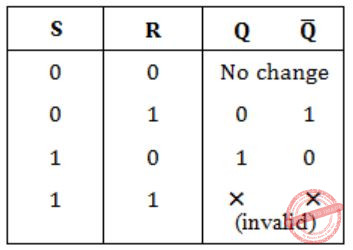So, Answer is Option (D).
 Question 19

If 73x (in base-x number system) is equal to 54y (in base-y number system), the possible values of x and y are

 A 8, 16 B 10, 12 C 9, 13 D 8, 11
Digital-Logic-Design       Number-Systems
Question 19 Explanation:
(73)x = (54)y
7x+3 = 5y+4
7x-5y = 1
Only option (D) satisfies above equation.
 Question 20

Which of the following addressing modes are suitable for program relocation at run time?

```(i)  Absolute addressing     (ii) Based addressing
(iii) Relative addressing     (iv) Indirect addressing ```
 A (i) and (iv) B (i) and (ii) C (ii) and (iii) D (i), (ii) and (iv)
Computer-Organization       Addressing-Modes
Question 20 Explanation:
Absolute Addressing:
A fixed address in memory which indicates a location by specifying a distance from another location. In this displacement type addressing is preferred.
So, option A is false.
Based Addressing:
This scheme is used by computers to control access to memory. In this pointers are replaced by protected objects which can be executed by kernel (or) some other privileged process authors.
So, this is suitable for program relocation at runtime.
Relative Addressing:
The offset of the relative addressing is to allow reference to code both before and after the instruction.
This is also suitable.
Indirect Addressing:
Which leads to extra memory location which can be not suitable at run time.
This is not suitable.
→ Only Based Addressing and Relative Addressing are suitable.
 Question 21

he minimum number of page frames that must be allocated to a running process in a virtual memory environment is determined by

 A the instruction set architecture B page size C physical memory size D number of processes in memory
Operating-Systems       Virtual Memory
Question 21 Explanation:
→ Based on Instruction Set Architecture each process can be need minimum no. of pages.
→ An ISA permits multiple implementations that may vary in performance, physical size and monetary cost.
 Question 22

How many 8-bit characters can be transmitted per second over a 9600 baud serial communication link using asynchronous mode of transmission with one start bit, eight data bits, two stop bits, and one parity bit?

 A 600 B 800 C 876 D 1200
Computer-Networks       Serial-Communication
Question 22 Explanation:
In Serial port communication baud rate = bit rate.
So bit rate is 9600 bps.
To send one char we need to send (1 + 8 + 2 +1) = 12
So total char send = 9600 / 12 = 800
 Question 23

Identify the correct translation into logical notation of the following assertion.

`      "Some boys in the class are taller than all the girls"  `

Note: taller(x,y) is true if x is taller than y.

 A (∃x) (boy(x) → (∀y) (girl(y) ∧ taller(x,y))) B (∃x) (boy(x) ∧ (∀y) (girl(y) ∧ taller(x,y))) C (∃x) (boy(x) → (∀y) (girl(y) → taller(x,y))) D (∃x) (boy(x) ∧ (∀y) (girl(y) → taller(x,y)))
Engineering-Mathematics       Propositional-Logic
Question 23 Explanation:
Don't confuse with '∧' and '→'
'∧' → predicts statements are always true, no matter the value of x.
'→' → predicts there is no need of left predicate to be true always, but whenever it becomes true, then right predicate must be true.
Option D:
There exists a some boys who are taller than of all girls y.
 Question 24

Consider the binary relation:

`       S = {(x,y)|y = x+1 and x,y ∈ {0,1,2, ...}}  `

The reflexive transitive closure of S is

 A {(x, y)|y > x and x, y ∈ {0, 1, 2, ... }} B {(x, y)|y ≥ x and x, y ∈ {0, 1, 2, ... }} C {(x, y)|y < x and x, y ∈ {0, 1, 2, ... }} D {(x, y)|y ≤ x and x, y ∈ {0, 1, 2, ... }}
Engineering-Mathematics       Sets-And-Relation
Question 24 Explanation:
Transitive means that x is related to greater value y and reflexive means x related to y.
Answer is option B.
{(x, y)|y ≥ x and x, y ∈ {0, 1, 2, ... }}
 Question 25

If a fair coin is tossed four times. What is the probability that two heads and two tails will result?

 A 3/8 B 1/2 C 5/8 D 3/4
Engineering-Mathematics       Probability
Question 25 Explanation:
A fair coin is tossed 4 times.
Then total number of possibilities = 24 = 16
No. of possibilities getting 2 heads and 2 tails is
HHTT, HTHT, TTHH, THTH, THHT, HTTH = 6
Probability of getting 2 heads and 2 tails is
= No. of possibilities/Total no. of possibilities = 6/16 = 3/8
 Question 26

The number of different n × n symmetric matrices with each element being either 0 or 1 is: (Note: power(2,x) is same as 2x)

 A power (2,n) B power (2,n2) C power (2, (n2 + n)/2) D power (2, (n2 - n)/2)
Engineering-Mathematics       Linear-Algebra
Question 26 Explanation:
If a matrix is symmetric then
A[i][j] = A[j][i]
So, we have only two choices, they are either upper triangular elements (or) lower triangular elements.
No. of such elements are
n + (n-1) + (n-2) + ... + 1
n(n+1)/2
We have two choices, thus we have
2(n(n+1)/2) = 2((n2+n)/2) choices
i.e., Power (2, (n2+n)/2).
 Question 27

Let A, B, C, D be n × n matrices, each with non-­zero determinant. If ABCD = 1, then B-1 is

 A D-1C-1A-1 B CDA C ADC D Does not necessarily exist
Engineering-Mathematics       Linear-Algebra
Question 27 Explanation:
ABCD are n × n matrices with non-zero determinant.
ABCD = I
Pre multiply A-1 on both sides
A-1ABCD = A-1⋅I
BCD = A-1
Pre multiply B-1 on both sides
B-1BCD = B-1A-1
CD = B-1A-1
Post multiply A on both sides
CDA = B-1A-1⋅A
∴ CDA = B-1(I)
∴ CDA = B-1
 Question 28

What is the result of evaluating the following two expressions using three-digit floating point arithmetic with rounding?

```   (113. + -111.) + 7.51
113. + (-111. + 7.51) ```
 A 9.51 and 10.0 respectively B 10.0 and 9.51 respectively C 9.51 and 9.51 respectively D 10.0 and 10.0 respectively
Digital-Logic-Design       Number-Systems
Question 28 Explanation:
(113. + -111.) + 7.51
= (2) + 7.51
= 9.51 (✔️)
113. + (-111. + 7.51)
= 113. + (-103.51)
= 113. + -103
= 10 (✔️)
 Question 29

The tightest lower bound on the number of comparisons, in the worst case, for comparison-based sorting is of the order of

 A n B n2 C nlogn D nlog2n
Algorithms        Time-Complexity
Question 29 Explanation:
In comparison based sorting, the tightest bound of number of comparisons is θ(n log n).
→ Tightest upper bound is (big O).
Tightest lower bound is (big Ω).
 Question 30

The problems 3-SAT and 2-SAT are

 A both in P B both NP complete C NP-complete and in P respectively D undecidable and NP-complete respectively
Algorithms        P-NP
Question 30 Explanation:
SAT → Boolean satisfiability problem & it is a decision problem.
2-SAT and 3-SAT is a NP-complete.
 Question 31

Consider the following C function:

```     int f(int n)
{
static int i = 1;
if (n >= 5)
return n;
n = n+i;
i++;
return f(n);
} ```

The value returned by f(1) is

 A 5 B 6 C 7 D 8
Programming       C-Programming
Question 31 Explanation:The value return by f(1) = 7
 Question 32

Consider the following program fragment for reversing the digits in a given integer to obtain a new integer. Let n = d1d2…dm.

```     int n, rev;
rev = 0;
while (n > 0)
{
rev = rev*10 + n%10;
n = n/10;
}```

The loop invariant condition at the end of the ith iteration is:

 A n = d1d2…dm-i and rev = dmdm-1…dm-i+1 B n = dm-i+1…dm-1dm or rev = dm-i…d2d1 C n ≠ rev D n = d1d2…dm and rev = dm…d2d1
Programming       Loop-Invariants
Question 32 Explanation:
In each iteration the right most digit of n is going to make to the right end of the reverse.
 Question 33

Consider the following C program segment:

```     char p;
char *s = "string";
int length = strlen(s);
int i;
for (i = 0; i < length; i++)
p[i] = s[length — i];
printf("%s",p); ```

The output of the program is

 A gnirts B string C gnirt D no output is printed
Programming       C-Programming
Question 33 Explanation:
Every string is to be end with '\0'.
P = S[7-1] = S = \0.
In P[ ], the first character is '\0'. Then it will results a empty string. If P become '\0', then it doesn't consider about next values in sequence.
 Question 34

It is desired to design an object-oriented employee record system for a company. Each employee has a name, unique id and salary. Employees belong to different categories and their salary is determined by their category. The functions to get Name, getld and compute salary are required. Given the class hierarchy below, possible locations for these functions are:

(i) getld is implemented in the superclass
(ii) getld is implemented in the subclass
(iii) getName is an abstract function in the superclass
(iv) getName is implemented in the superclass
(v) getName is implemented in the subclass
(vi) getSalary is an abstract function in the superclass
(vii) getSalary is implemented in the superclass
(viii) getSalary is implemented in the subclassChoose the best design

 A (i), (iv), (vi), (viii) B (i), (iv), (vii) C (i), (iii), (v), (vi), (viii) D (ii), (v), (viii)
Programming       OOPS
Question 34 Explanation:
Name and id are a property of every employee and independent of their category. So these should be implemented in superclass. Every employee has a salary but this is determined by their category. So getsalary must be abstract function in superclass and implemented in subclass.
 Question 35

Consider the label sequences obtained by the following pairs of traversals on a labeled binary tree. Which of these pairs identify a tree uniquely?

```(i) preorder and postorder     (ii) inorder and postorder
(iii) preorder and inorder     (iv) level order and postorder ```
 A (i) only B (ii), (iii) C (iii) only D (iv) only
Data-Structures       Binary-Trees
Question 35 Explanation:
→ We have some combinations such that which can be able to identity a tree
(i) Inorder and Preorder
(ii) Inorder and Postorder
(iii) Inorder and Levelorder
→ And following are do not
(i) Post order and Preorder
(ii) Pre order and Level order
(iii) Post order and Level order
 Question 36

A circularly linked list is used to represent a Queue. A single variable p is used to access the Queue. To which node should p point such that both the operations enQueue and deQueue can be performed in constant time?A rear node B front node C not possible with a single pointer D node next to front
Data-Structures       Linked-List
Question 36 Explanation:
We can perform enqueue and dequeue from rear within O(1) time. But from the front node we cannot rear from front in O(1) time.
 Question 37

The elements 32, 15, 20, 30, 12, 25, 16 are inserted one by one in the given order into a Max Heap. The resultant Max Heap is

 ABC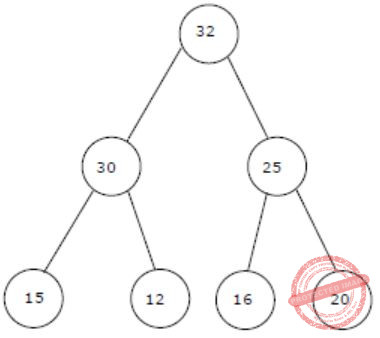DData-Structures       Heap-Tree
Question 37 Explanation:
32, 15, 20, 30, 12, 25, 16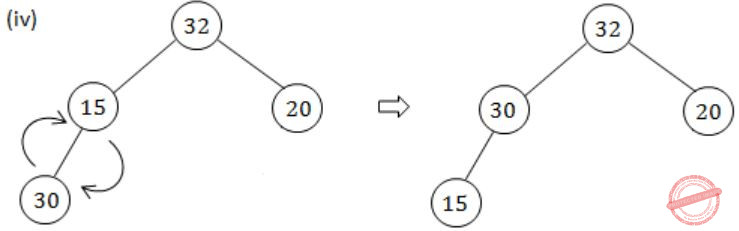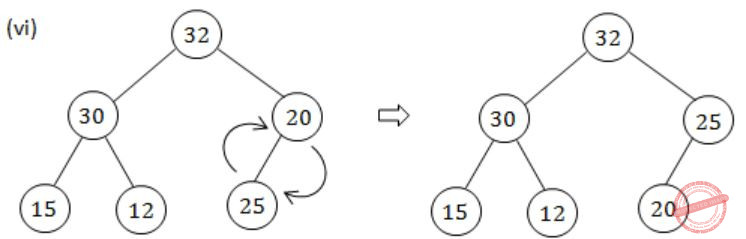Question 38
Assume that the operators +, -, × are left associative and ^ is right associative. The order of precedence (from highest to lowest) is ^, x , +, -. The postfix expression corresponding to the infix expression a + b × c - d ^ e ^ f is
 A abc×+def^^- B abc×+de^f^- C ab+c×d-e^f^ D -+a×bc^^def
Data-Structures       Stacks
Question 38 Explanation:
a+b×c-d^e^f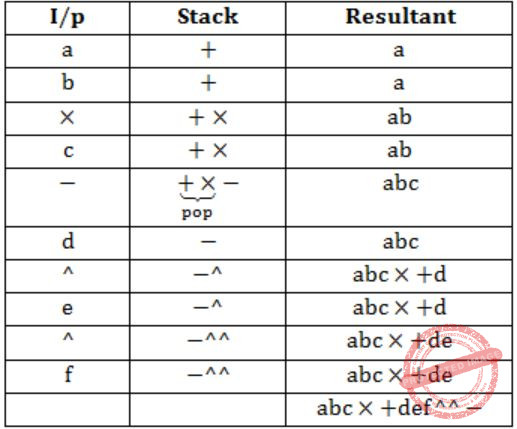Note: When low precedence operator enters into stack then pop.
 Question 39

Two matrices M1 and M2 are to be stored in arrays A and B respectively. Each array can be stored either in row-major or column-major order in contiguous memory locations. The time complexity of an algorithm to compute M1 × M2 will be

 A best if A is in row-major, and B is in column major order B best if both are in row-major order C best if both are in column-major order D independent of the storage scheme
Algorithms        Time-Complexity
Question 39 Explanation:
Running time of an algorithm is always independent of the storage scheme. While computing the running time of an algorithm we assume that to access any element time taken is same. So answer is (D).
But if the question would have asked best time complexity in which of the following implementation (not algorithm) then Option (A) is correct.
 Question 40

Suppose each set is represented as a linked list with elements in arbitrary order. Which of the operations among union, intersection, membership, cardinality will be the slowest?

 A union only B intersection, membership C membership, cardinality D union, intersection
Data-Structures       Linked-List
Question 40 Explanation:
Let no. of elements in list 1 be n1.
Let no. of elements in list 2 be n2.
Union:
To union two lists, for each element in one list we will search in other list, to avoid duplicates. So, time complexity will be O(n1×n2).
Intersection:
To take intersection of two lists, for each element in one list we will search in other list if it is present or not. So time complexity will be O(n1 × n2).
Membership:
In this we search if particular element is present in the list or not. So time complexity will be O(n1 + n2).
Cardinality:
In this we find the size of set or list. So to find size of list we have to traverse each list once. So time complexity will be O(n1+n2).
Hence, Union and Intersection will be slowest.
 Question 41

Consider the following C program

```     main()
{   int x, y, m, n;
scanf ("%d %d", &x, &y);
/* Assume x > 0 and y > 0  */
m = x; n = y;
while (m! = n)
{    if (m > n)
m = m - n;
else
n = n - m;
}
print f ("% d", n);
}```

The program computes

 A x + y using repeated subtraction B x mod y using repeated subtraction C the greatest common divisor of x and y D the least common multiple of x and y
Programming       C-Programming
Question 41 Explanation:
Given code is same as Euclid's Algorithm for finding Greatest Common Divisor(GCD).
 Question 42

What does the following algorithm approximate?(Assume m > 1, ε > 0).

```     x = m;
y = 1;
while (x - y > ε)
{          x = (x + y)/2;
y = m/x;
}
print(x); ```
 A log m B m2 C m1/2 D m1/3
Algorithms        Identify-Function
Question 42 Explanation:
Put y = m/x in x = (x+y)/2
x = (x + m/x)/2
2x = x2+m/x
2x2 = x2 + m
x2 = m
x = √m = m1/2
 Question 43

Consider the following C program segment

```struct CellNode
{
struct CelINode *leftchild;
int element;
struct CelINode *rightChild;
}

int Dosomething(struct CelINode *ptr)
{
int value = 0;
if (ptr != NULL)
{
if (ptr->leftChild != NULL)
value = 1 + DoSomething(ptr->leftChild);
if (ptr->rightChild != NULL)
value = max(value, 1 + DoSomething(ptr->rightChild));
}
return (value);
}```

The value returned by the function DoSomething when a pointer to the root of a non-empty tree is passed as argument is

 A The number of leaf nodes in the tree B The number of nodes in the tree C The number of internal nodes in the tree D The height of the tree
Data-Structures       Binary-Trees
Question 43 Explanation:
→ Dosomething ( ) returns the max (height of left child + 1, height right child + 1).
→ So given that pointer to root of tree is passed to DoSomething ( ), it will return height of the tree. It is done when the height of single node is '0'.
 Question 44

Suppose we run Dijkstra’s single source shortest-path algorithm on the following edge weighted directed graph with vertex P as the source. In what order do the nodes get included into the set of vertices for which the shortest path distances are finalized?A P, Q, R, S, T, U B P, Q, R, U, S, T C P, Q, R, U, T, S D P, Q, T, R, U, S
Algorithms        Shortest-Path
Question 44 Explanation:P, Q, R, U, S, T
 Question 45

Consider the grammar with the following translation rules and E as the start symbol.

```E → E1 # T     {E.value = E1.value * T.value}
|    T     {E.value = T.value}
T → T1 & F     {T.value = T1.value + F.value}
|    F     {T.value = F.value}
F → num        {F.value = num.value}  ```

Compute E.value for the root of the parse tree for the expression: 2 # 3 & 5 # 6 & 4.

 A 200 B 180 C 160 D 40
Compiler-Design       Grammar
Question 45 Explanation:
Given expression is
2 # 3 & 5 # 6 & 4
→ Here # means multiplication (*)
& means addition (+)
→ & is having more precedence because it is far from starting symbol, here # and & are left associatives.
2 # 3 & 5 # 6 & 4
⇒ (2 * (3+5)) * (6+4)
⇒ (2 * 8) * (10)
⇒ 16 * 10 = 160
 Question 46

Consider the following set of processes, with the arrival times and the CPU-burst times given in milliseconds.

```  Process   Arrival Time    Burst Time
P1          0              5
P2          1              3
P3          2              3
P4          4              1 ```

What is the average turnaround time for these processes with the preemptive shortest remaining processing time first (SRPT) algorithm?

 A 5.5 B 5.75 C 6 D 6.25
Operating-Systems       Process-Scheduling
Question 46 Explanation:
Uses SRPT Algorithm:Avg. TAT = 12+3+6+1/4 = 22/4 = 5.50
 Question 47

Consider a system with a two-level paging scheme in which a regular memory access takes 150 nanoseconds, and servicing a page fault takes 8 milliseconds. An average instruction takes 100 nanoseconds of CPU time, and two memory accesses. The TLB hit ratio is 90%, and the page fault rate is one in every 10,000 instructions. What is the effective average instruction execution time?

 A 645 nanoseconds B 1050 nanoseconds C 1215 nanoseconds D 2060 nanoseconds
Operating-Systems       Virtual Memory
Question 47 Explanation:
Effective average instruction time = CPU time + 2 EMAT
= 100ns + 2EMAT
Now lets calculate EMAT,
EMAT = TLB + miss rate × 2 × 150ns + 150ns + 1/10000 × 8ms
= 0 + 0.1 × 300ns + 150ns + 800ns
= 980ns
∴ Effective average instruction time,
= 100ns + 2 × 980ns
= 2060ns
 Question 48

Consider two processes P1 and P2 accessing the shared variables X and Y protected by two binary semaphores SX and SY respectively, both initialized to 1. P and V denote the usual semaphone operators, where P decrements the semaphore value, and V increments the semaphore value. The pseudo-code of P1 and P2 is as follows:

```P1 :
While true do {
L1 : ................
L2 : ................
X = X + 1;
Y = Y - 1;
V(SX);
V(SY);
}
P2 :
While true do {
L3 : ................
L4 : ................
Y = Y + 1;
X = Y - 1;
V(SY);
V(SX);
}```

In order to avoid deadlock, the correct operators at L1, L2, L3 and L4 are respectively

 A P(Sy), P(Sx); P(Sx), P(Sy) B P(Sx), P(Sy); P(Sy), P(Sx) C P(Sx), P(Sx); P(Sy), P(Sy) D P(Sx), P(Sy); P(Sx), P(Sy)
Operating-Systems       Process-Synchronization
Question 48 Explanation:
Option D:
P1 : line 1
P2 : line 3 (block require Sx)
P1 : line 2
P2 : line 4 (still in block state)
P1 : execute CS, the push up the value of Sx.
P2 : line 3 line 4 (block require Sy)
P1 : push up Sy
P2 : line 4 get Sy and enter into CS
P2 : start execution.
So option D is Answer.
 Question 49

A Unix-style i-node has 10 direct pointers and one single, one double and one triple indirect pointers. Disk block size is 1 Kbyte, disk block address is 32 bits, and 48-bit integers are used. What is the maximum possible file size?

 A 224 bytes B 232 bytes C 234 bytes D 248 bytes
Operating-Systems       Disk-Scheduling
Question 49 Explanation:
Maximum size of file = 10 Direct + 1 Single Indirect + 1 Double Indirect + 1 Triple Indirect
= 10 + 28 + (28)2 + (28)3
= 224 Blocks (App)
Size of each block = 210
Maximum file size = 224 × 210 = 234
 Question 50

The relation scheme Student Performance (name, courseNo, rollNo, grade) has the following functional dependencies:

```   name, courseNo → grade
rollNo, courseNo → grade
name → rollNo
rollNo → name ```

The highest normal form of this relation scheme is

 A 2 NF B 3 NF C BCNF D 4NF
Database-Management-System       Normalization
Question 50 Explanation:
Student Performance (name, courseNo, rollNo, grade)
name, courseNo  → grade →(I)
rollNo, courseNo → grade →(II)
name → rollNo →(III)
rollNo → name →(IV)
Candidate keys: name, courseNo (or) rollNo
Its is not BCNF, because the relation III, there is no relationship from super key.
name → rollNo
It is not BCNF, name is not super key.
It belongs to 3NF, because if X→Y, Y is prime then it is in 3NF.
 Question 51

Consider the relation Student (name, sex, marks), where the primary key is shown underlined, pertaining to students in a class that has at least one boy and one girl. What does the following relational algebra expression produce? (Note: p is the rename operator).The condition in join is "(sex = female ^ x = male ^ marks ≤ m)"

 A names of girl students with the highest marks B names of girl students with more marks than some boy student C names of girl students with marks not less than some boy students D names of girl students with more marks than all the boy students
Database-Management-System       Relational-Algebra
Question 51 Explanation:
In the operator '-' between the two subexpression gives the names of all female students whose marks are greater than the all the male students of the class.
 Question 52

The order of an internal node in a B+ tree index is the maximum number of children it can have. Suppose that a child pointer takes 6 bytes, the search field value takes 14 bytes, and the block size is 512 bytes. What is the order of the internal node?

 A 24 B 25 C 26 D 27
Database-Management-System       B+-Trees
Question 52 Explanation:
Block size = (n-1) * key size + n * child pointer
Child pointer = 6 bytes
key size = 14 bytes
Block size = 512 bytes
⇒ 512 = (n-1)14 + n(6)
512 = 20n - 14
n = 512+14/20 = 526/20 = 26.3
∴ n = 26
 Question 53

The employee information in a company is stored in the relation

```Employee (name, sex, salary, deptName)
Consider the following SQL query
Select deptName
From Employee
Where sex = 'M'
Group by deptName
Having avg (salary) >
(select avg (salary) from Employee)```

It returns the names of the department in which

 A the average salary is more than the average salary in the company B the average salary of male employees is more than the average salary of all male employees in the company C the average salary of male employees is more than the average salary of employees in the same department D the average salary of male employees is more than the average salary in the company
Database-Management-System       SQL
Question 53 Explanation:
Group by (avg(salary) > (select avg (salary) from employee))
This results the employees who having the salary more than the average salary.
Sex = M
Selects the Male employees whose salary is more than the average salary in the company.
 Question 54

A and B are the only two stations on an Ethernet. Each has a steady queue of frames to send. Both A and B attempt to transmit a frame, collide, and A wins the first backoff race. At the end of this successful transmission by A, both A and B attempt to transmit and collide. The probability that A wins the second backoff race is:

 A 0.5 B 0.625 C 0.75 D 1
Computer-Networks       Ethernet
Question 54 Explanation:
A has 5 chances to win out of 8 combinations.
The probability that A wins the second back-off race = 5/8 = 0.625
More explanation in the video.
 Question 55

The routing table of a router is shown below:

``` Destination     Sub net mask 	     Interface
128.75.43.0 	 255.255.255.0 	        Eth0
128.75.43.0 	 255.255.255.128 	Eth1
192.12.17.5 	 255.255.255.255 	Eth3
Default 	  	                Eth2 ```

On which interfaces will the router forward packets addressed to destinations 128.75.43.16 and 192.12.17.10 respectively?

 A Eth1 and Eth2 B Eth0 and Eth2 C Eth0 and Eth3 D Eth1 and Eth3
Computer-Networks       IP-Address
Question 55 Explanation:
Router decides route for packet by ANDing subnet mask and IP address.
If results of ANDing subnet masks and IP address are same then subnet mask with higher number of 1s is preferred.
IP address 128.75.43.16 is AND with 255.255.255.0 results 128.75.43.0 Net ID which is similar to destination of this mask, but ANDing 128.75.43.16 with 255.255.255.128 also results same destination. So, here, mask with higher number of one is considered and router will forward packet to Eth1.
ANDing 192.12.17.10 with three subnet mask in table does not result in destination Net ID so router will forward this packet to default network via Eth2.
 Question 56

Consider three IP networks A, B and C. Host HA in network A sends messages each containing 180 bytes of application data to a host HC in network C. The TCP layer prefixes a 20 byte header to the message. This passes through an intermediate net­work B. The maximum packet size, including 20 byte IP header, in each network is:

```     A : 1000 bytes
B : 100 bytes
C : 1000 bytes```

The network A and B are connected through a 1 Mbps link, while B and C are connected by a 512 Kbps link (bps = bits per second).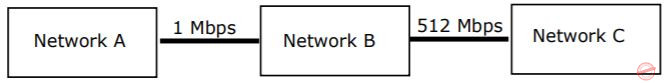Assuming that the packets are correctly delivered, how many bytes, including headers, are delivered to the IP layer at the destination for one application message, in the best case? Consider only data packets.

 A 200 B 220 C 240 D 260
Computer-Networks       IPv4-and-TCP
Question 56 Explanation:
Application data is 180 bytes. TCP layer add 20 bytes to it and passes to IP layer, data for IP layer becomes 200 byte. HA send packet by adding 20 byte of IP header. So total size of IP packet is 220 bytes. Since, maximum packet size for packet in network A is 1000 bytes, there will be no fragmentation at network A. IP Layer at Network B removes IP header and receive 200 bytes of data. Network B has Maximum packet size 100 bytes including 20 byte IP header, network B divide data in 80 bytes fragments and add 20 byte of IP header to it.
Data will be divided in three packets as:
First packet: 80 bytes + 20 byte of header
Second packet: 80 bytes + 20 byte of header
Third packet: 40 bytes + 20 byte of header
Note: Defragmentation (grouping of fragments) is done only at destination.
HC will receive total 260 bytes including header.
 Question 57

Consider three IP networks A, B and C. Host HA in network A sends messages each containing 180 bytes of application data to a host HC in network C. The TCP layer prefixes a 20 byte header to the message. This passes through an intermediate net­work B. The maximum packet size, including 20 byte IP header, in each network is:

```     A : 1000 bytes
B : 100 bytes
C : 1000 bytes```

The network A and B are connected through a 1 Mbps link, while B and C are connected by a 512 Kbps link (bps = bits per second).What is the rate at which application data is transferred to host HC? Ignore errors, acknowledgements, and other overheads.

 A 325.5 Kbps B 354.5 Kbps C 409.6 Kbps D 512.0 Kbps
Computer-Networks       IPv4-and-TCP
Question 57 Explanation:
HC will receive 260 bytes in which only 180 bytes are of application data.
Application data is transferred at rate of (180/260) x 512 Kbps = 354.46 Kbps
 Question 58

A circuit outputs a digit in the form of 4 bits. 0 is represented by 0000, 1 by 0001, ..., 9 by 1001. A combinational circuit is to be designed which takes these 4 bits as input and outputs 1 if the digit ≥ 5, and 0 otherwise. If only AND, OR and NOT gates may be used, what is the minimum number of gates required?

 A 2 B 3 C 4 D 5
Digital-Logic-Design       Combinational-Circuits
Question 58 Explanation:= A + BD + BC
= A + B (D + C)
So minimum two OR gates and 1 AND gate is required. Hence, in total minimum 3 gates is required.
 Question 59

Which are the essential prime implicants of the following Boolean function?

` f(a,b,c) = aȼc + acȼ + bȼc `
 A aȼc and acȼ B aȼc and bȼc C aȼc only D acȼ and bcȼ
Digital-Logic-Design       K-Map
Question 59 Explanation:
From given function 'f' we can draw,There are two EPI,
A'C and AC'.
 Question 60

Consider a multiplexer with X and Y as data inputs and Z as control input. Z = 0 selects input X, and Z = 1 selects input Y. What are the connections required to realize the 2-variable Boolean function f = T + R, without using any additional hardware?

 A R to X, 1 to Y, T to Z B T to X, R to Y, T to Z C T to X, R to Y, 0 to Z D R to X, 0 to Y, T to Z
Digital-Logic-Design       Multiplexer
Question 60 Explanation:
Given,f = z'x + zy
Put z=T, x=R, y=1 in f
f = T'R + T = (T+T') (R+T) = T+R
Hence, correct option is (A).
 Question 61

Consider the partial implementation of a 2-bit counter using T flip-flops following the sequence 0-2-3-1-0, as shown below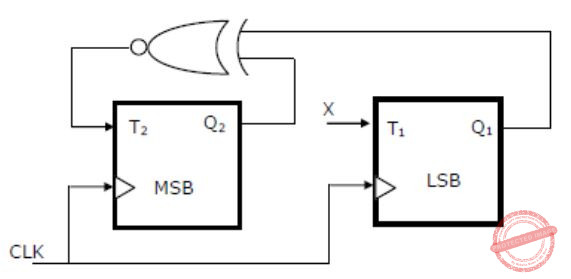To complete the circuit, the input X should be

 A Q2c B Q2 + Q1 C (Q1 + Q2)c D Q1 ⊕ Q2
Digital-Logic-Design       Sequential-Circuits
Question 61 Explanation:
Sequence given is
0 - 2 - 3 - 1 - 0
or
00 - 10 - 11 - 01 - 00
From the given sequence, we have state table as,Now we have present state and next state, so we should use excitation table of T flip-flop,From state table,
T2 = Q2⊙Q1 and T1 = Q2⊕Q1
X = T1 = Q2⊕Q1
 Question 62

A 4-bit carry lookahead adder, which adds two 4-bit numbers, is designed using AND, OR, NOT, NAND, NOR gates only. Assuming that all the inputs are available in both complemented and uncomplemented forms and the delay of each gate is one time unit, what is the overall propagation delay of the adder? Assume that the carry network has been implemented using two-level AND-OR logic.

 A 4 time units B 6 time units C 10 time units D 12 time units
Digital-Logic-Design       Carry-Look-Ahead-Adder
Question 62 Explanation:
The 4-bit addition will be calculated in 3 stages:
1) (2 time units) In 2 time units we can compute Gi and Pi in parallel, 2 time units for Pi since its an XOR operation and 1 time unit for Gi since its an AND operation.
2) (2 time units) Once Gi and Pi are available, we can calculate the carries, Ci, in 2 time units.
Level-1 we can compute all the conjunctions (AND).
Example: P3G2, P3P2G1, P3P2P1G0 and P3P2P1P0C0 which are required for C4.
Level-2 we get the carries by computing the disjunction (OR).
3) (2 time units) Finally, we compute the sum in 2 time units, as its a XOR operation.
Hence, the total is 2+2+2 = 6 time units.
 Question 63

Consider the following program segment for a hypothetical CPU having three user registers R1, R2 and R3.

``` Instruction 	     Operation 	      Instruction Size(in words)
MOV R1,5000; 	 ;R1 ← Memory 	         2
MOV R2(R1); 	 ;R2 ← Memory[(R1)] 	         1
ADD R2,R3; 	 ;R2 ← R2 + R3 	                 1
MOV 6000,R2; 	 ;Memory ← R2 	         2
HALT 	         ;Machine halts 	         1 ```

Consider that the memory is byte addressable with size 32 bits, and the program has been loaded starting from memory location 1000 (decimal). If an interrupt occurs while the CPU has been halted after executing the HALT instruction, the return address (in decimal) saved in the stack will be

 A 1007 B 1020 C 1024 D 1028
Computer-Organization       Machine-Instructions
Question 63 Explanation:
Byte addressable with size = 32 bits (word size) = 4 bytes
→ Interrupt occurs after executing halt instruction
So, number of instructions = 2+1+1+2+1 = 7
→ Each instruction with 4 bytes, then total instruction size = 7 * 4 = 28
→ Memory start location = 1000
→ Instruction saved location = 1000 + 28 = 1028
1028 is the starting address of next instruction.
 Question 64

Consider the following program segment for a hypothetical CPU having three user registers R1, R2 and R3.

``` Instruction 	     Operation 	      Instruction Size(in words)
MOV R1,5000; 	 ;R1 ← Memory 	         2
MOV R2(R1); 	 ;R2 ← Memory[(R1)] 	         1
ADD R2,R3; 	 ;R2 ← R2 + R3 	                 1
MOV 6000,R2; 	 ;Memory ← R2 	         2
HALT 	         ;Machine halts 	         1 ```

Let the clock cycles required for various operations be as follows:

```Register to/ from memory transfer     : 3 clock cycles
ADD with both operands in register    : 1 clock cycle
Instruction fetch and decode          : 2 clock cycles per word  ```

The total number of clock cycles required to execute the program is

 A 29 B 24 C 23 D 20
Computer-Organization       Machine-Instructions
Question 64 Explanation:Question 65

Consider a small two-way set-associative cache memory, consisting of four blocks. For choosing the block to be replaced, use the least recently used (LRU) scheme. The number of cache misses for the following sequence of block addresses is 8, 12, 0, 12, 8

 A 2 B 3 C 4 D 5
Computer-Organization       Cache
Question 65 Explanation:
Cache consists of 4 blocks and is 2-way set associative. So cache have 2 sets each of size 2 blocks.
The given sequence is 8, 12, 0, 12, 8.So in total 4 misses is there.
 Question 66

Let A = 1111 1010 and B = 0000 1010 be two 8-bit 2's complement numbers. Their product in 2's complement is

 A 1100 0100 B 1001 1100 C 1010 0101 D 1101 0101
Digital-Logic-Design       Number-Systems
Question 66 Explanation:
A = 1111 1010 = -610 [2's complement number]
B = 0000 1010 = 1010 [2's complement number]
A×B = -6×10 = - 6010
⇒ -6010 = 101111002
= 110000112 (1's complement)
= 110001002 (2's complement)
 Question 67

The microinstructions stored in the control memory of a processor have a width of 26 bits. Each microinstruction is divided into three fields: a micro-operation field of 13 bits, a next address field (X), and a MUX select field (Y). There are 8 status bits in the inputs of the MUX.How many bits are there in the X and Y fields, and what is the size of the control memory in number of words?

 A 10, 3, 1024 B 8, 5, 256 C 5, 8, 2048 D 10, 3, 512
Computer-Organization       Micro-programming
Question 67 Explanation:
Processed width = 26 bits
Micro process field width = 13 bits
MUX consists of 8 state bits, then it require 3 inputs to select input line.
No. of bits for next address field = 26 - 13 - 3= 10
For 10 bit addressing, we require 210 memory size
Size (X, Y) = 10, 3
 Question 68

A hard disk with a transfer rate of 10 Mbytes/ second is constantly transferring data to memory using DMA. The processor runs at 600 MHz, and takes 300 and 900 clock cycles to initiate and complete DMA transfer respectively. If the size of the transfer is 20 Kbytes, what is the percentage of processor time consumed for the transfer operation?

 A 5.0% B 1.0% C 0.5% D 0.1%
Computer-Organization       DMA
Question 68 Explanation:
Clock cycle time = 1/600 × 10-6 (time = 1/frequency)
For DMA initiation and completion total 1200 cycles is required. So total time will be = 1200 × 1/600 × 10-6 = 2μs
Disk transfer rate = 10MB/s
1B = 1/107 s
So, total transfer 20KB time taken will be,
20 × 103 × 1/(107) s
= 2000μs
∴ Percentage of processor time consumed is,
2/2+2000 × 100 = 0.1%
 Question 69

A 4-stage pipeline has the stage delays as 150, 120, 160 and 140 nanoseconds respectively. Registers that are used between the stages have a delay of 5 nanoseconds each. Assuming constant clocking rate, the total time taken to process 1000 data items on this pipeline will be

 A 120.4 microseconds B 160.5 microseconds C 165.5 microseconds D 590.0 microseconds
Computer-Organization       Pipelining
Question 69 Explanation:
First instruction will take complete four cycle for execution. And then after that all 999 instruction will take only 1 cycle for execution to be completed. So time required to process 1000 instruction or data items is,
1st instruction × 4 × clock time + 999 instruction × 1 × clock time
1 × 4 × 165ns + 999 × 1 × 165ns
= 1654.95ns
= 165.5μs
 Question 70

The following propositional statement is (P → (Q v R)) → ((P v Q) → R)

 A satisfiable but not valid B valid C a contradiction D None of the above
Engineering-Mathematics       Propositional-Logic
Question 70 Explanation:
(P→(Q∨R)) → ((P∨Q)→R)
If P=T; Q=T; R=T
(P→(T∨T)) → ((T∨T)→R)
(P→T) → (T→R)
(T→T) → (T→T)
T→T
T(Satisfiable)
 Question 71

How many solutions does the following system of linear equations have?

```  -x + 5y = -1
x - y = 2
x + 3y = 3 ```
 A infinitely many B two distinct solutions C unique D none
Engineering-Mathematics       Linear-Algebra
Question 71 Explanation:rank = r(A) = r(A|B) = 2
rank = total no. of variables
Hence, unique solution.
 Question 72

The following is the incomplete operation table a 4-element group.The last row of the table is

 A c a e b B c b a e C c b e a D c e a b
Engineering-Mathematics       Sets-And-Relation
Question 72 Explanation:The last row is c e a b.
 Question 73

The inclusion of which of the following sets into

`S = {{1,2}, {1,2,3}, {1,3,5}, (1,2,4), (1,2,3,4,5}}`

is necessary and sufficient to make S a complete lattice under the partial order defined by set containment?

 A {1} B {1}, {2, 3} C {1}, {1, 3} D {1}, {1, 3}, (1, 2, 3, 4}, {1, 2, 3, 5}
Engineering-Mathematics       Sets-And-Relation
Question 73 Explanation:
A lattice is complete if every subset of partial ordered set is to be a supremum and infimum element.
For the set {1, 2, 3, 4, 5} there is no supremum element i.e., {1}.
Then clearly we need to add {1}, then it is to be a lattice.
 Question 74

An examination paper has 150 multiple-choice questions of one mark each, with each question having four choices. Each incorrect answer fetches -0.25 mark. Suppose 1000 students choose all their answers randomly with uniform probability. The sum total of the expected marks obtained by all these students is:

 A 0 B 2550 C 7525 D 9375
Engineering-Mathematics       Probability
Question 74 Explanation:
Probability of choosing a correct answer = 1/4
Probability of selecting a wrong answer = 3/4
For correct answer +1, for wrong answer-0.25;
Expected marks for each question = (1/4) × 1 + (3/4) -(0.25)
= 1/4 + (-3/16)
= 4-3/16
= 1/16
= 0.0625
Expected marks for 150 questions = 150 × 0.625 = 9.375
The sum total of expected marks obtained by 1000 students is = 1000×9.375 = 9375
 Question 75

Mala has a colouring book in which each English letter is drawn two times. She wants to paint each of these 52 prints with one of k colours, such that the colour-pairs used to colour any two letters are different. Both prints of a letter can also be coloured with the same colour. What is the minimum value of k that satisfies this requirement?

 A 9 B 8 C 7 D 6
Engineering-Mathematics       Combinatorics
Question 75 Explanation:
No. of letters from A-Z is = 26
Each is printed twice the no. of letters = 26×2 = 52
If Mala has k colours, she can have k pairs of same colours.
She also can have kC2 different pairs in which each pair is having different colours.
So total no. of pairs that can be coloured = k+kC2
k+kC2 ≥ 26
k+k(k-1)/2 ≥ 26
k(k+1)/2 ≥ 26
k(k+1) ≥ 52
k(k+1) ≥ 7*8
k≥7
 Question 76

In an M×N matrix such that all non-zero entries are covered in a rows and b columns. Then the maximum number of non-zero entries, such that no two are on the same row or column, is

 A ≤ a+b B ≤ max(a, b) C ≤ min(M-a, N-b) D ≤ min(a, b)
Engineering-Mathematics       Linear-Algebra
Question 76 Explanation:
Entry will be a member of same row and same column.
→ Such that a row can have maximum of a elements and no row has separate element and for b also same.
→ By combining the both, it should be ≤ (a,b).
 Question 77

The minimum number of colours required to colour the following graph, such that no two adjacent vertices are assigned the same colour, is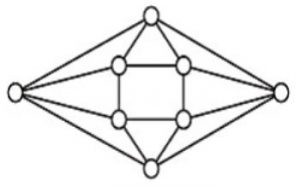A 2 B 3 C 4 D 5
Engineering-Mathematics       Graph-Theory
Question 77 Explanation:→ a, b, c, d = 4
→ The minimum no. of colours required to colour a graph = 4 (no two adjacent vertices have same colours)
 Question 78

Two n bit binary strings, S1 and S2, are chosen randomly with uniform probability. The probability that the Hamming distance between these strings (the number of bit positions where the two strings differ) is equal to d is

 A nCd /2n B nCd / 2d C d/2n D 1/2d
Engineering-Mathematics       Probability
Question 78 Explanation:
n binary bits with difference 'd' then no. of favourable cases = nCd
Total no. of cases where n positions have any binary bit = 2n
The probability of 'd' bits differ = nCd / 2n
 Question 79

How many graphs on n labeled vertices exist which have at least (n2 - 3n)/2 edges?

 AB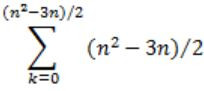C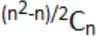D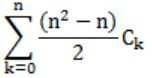Engineering-Mathematics       Graph-Theory
Question 79 Explanation:
No. of atleast edges = (n2-3n)/2 = e
Maximum no. of vertices = n(n-1)/2 = v
No. of graphs with minimum b edges is
= C(v,e) + C(v,e+1) + C(v,e+2) + ... + C(v,v)
= C((v,v-e) + C(v,v-(e+1)) + C(v,v-(e+2)) + ... + C(v,0)
= C(a,n) + C(a,n-1) + C(a,n-2) + ... + C(a,0) (since a-b=n)
= C(n(n-1)/2,n) + C(n(n-1)/2,n-1) + ... + C(n(n-1)/2,0)Question 80

A point is randomly selected with uniform probability in the X-Y plane within the rectangle with corners at (0,0), (1,0), (1,2) and (0,2). If p is the length of the position vector of the point, the expected value of p2 is

 A 2/3 B 1 C 4/3 D 5/3
Engineering-Mathematics       Probability
Question 80 Explanation:Above diagram shows the scenario of our question.
The length p of our position vector (x,y) is
p = √x2 + y2
p2 = x2 + y2
E(p2) = E(x2 + y2) = E(x2) + E(y2)
Now we need to calculate the probability density function of X and Y.
Since distribution is uniform,
X goes from 0 to 1, so PDF(x) = 1/1-0 = 1
Y goes from 0 to 2, so PDF(y) = 1/2-0 = 1/2
Now we evaluate,E(p2) = E(x2) + E(y2) = 5/3
 Question 81

Let G1 = (V,E1) and G2 = (V,E2) be connected graphs on the same vertex set V with more than two vertices. If G1 ∩ G2 = (V, E1 ∩ E2) is not a connected graph, then the graph G1 U G2 = (V, E1 U E2)

 A cannot have a cut vertex B must have a cycle C must have a cut-edge (bridge) D has chromatic number strictly greater than those of G1 and G2
Engineering-Mathematics       Graph-Theory
Question 81 Explanation:
Lets try to take counter example for each of them,
(A)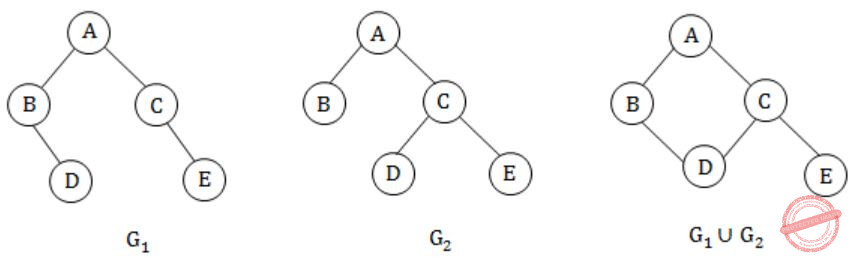False, since in G1∪G2 'C' is a cut vertex.
(B) True, for all conditions.
(C)False. G1∪G2 has no bridge.
D)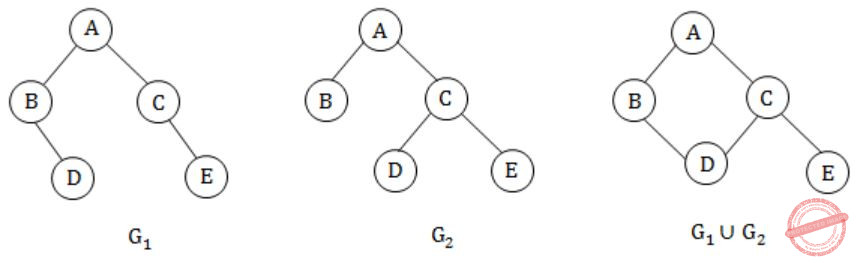False. G1∪G2, G1, G2 all the three graphs have chromatic number of 2.
 Question 82

Let A[1,...,n] be an array storing a bit (1 or 0) at each location, and f(m) is a function whose time complexity is θ(m). Consider the following program fragment written in a C like language:

```counter = 0;
for (i = 1; i < = n; i++)
{ if (A[i] == 1) counter++;
else { f(counter); counter = 0; }
}```

The complexity of this program fragment is

 A Ω(n2) B Ω(nlogn) and O(n2) C θ(n) D o(n)
Algorithms        Time-Complexity
Question 82 Explanation:
If the string contains all zero's then it takes O(n) time, because f(n) can call n times.
If the string contains all one's then it takes O(n) time, because counter++ can execute n times.
If it contains half 0's and half 1's then also it takes O(n) time.
 Question 83

The time complexity of the following C function is (assume n > 0)

```int recursive (int n) {
if (n == 1)
return (1);
else
return (recursive (n - 1) + recursive (n - 1));
} ```
 A O(n) B O(n log n) C O(n2) D O(2n)
Algorithms        Time-Complexity
Question 83 Explanation:
if (n==1)
return(1)
else
return(recursive(n-1) + recursive(n-1))
n>0:
T(2) = T(1) + T(1) = 2T(1)
T(3) = T(2) + T(2) = 2T(2) = 2⋅2T(1) = 22T(1)
T(4) = 23⋅T(1)

T(n) = 2n⋅T(1) = O(2n)
 Question 84

The recurrence equation

```   T(1) = 1
T(n) = 2T(n - 1) + n, n ≥ 2 ```

evaluates to

 A 2n+1 – n – 2 B 2n – n C 2n+1 – 2n – 2 D 2n + n
Algorithms        Recurrence
Question 84 Explanation:
T(1) =1
T(n) = 2T(n-1) + n
T(2) = 2T(1) + 2 = 2 + 2 = 4
T(3) = 2T(2) + n = 2(4) + 3 = 11
T(4) = 2T(3) + 4 = 22 + 4 = 26
Let check with the options:
Option A:
n=4
24+1 - 4 - 2
32 - 6
26 (✔️)
Option B:
n=4
2n-n
24-4
12(✖️)
Option C:
n=4
24+1 - 2(4) - 8
32 - 10
22(✖️)
Option D:
n=4
2n - n
24 - 4
12(✖️)
 Question 85

A program takes as input a balanced binary search tree with n leaf nodes and computes the value of a function g(x) for each node x. If the cost of computing g(x) is min{no. of leaf-nodes in left-subtree of x, no. of leaf-nodes in right-subtree of x} then the worst-case time complexity of the program is

 A Θ (n) B Θ (n log n) C Θ (n2) D Θ (n2 log n)
Algorithms        Time-Complexity
Question 85 Explanation:
Contains a balanced binary search tree.
⇒ g(x) = min (no. of leaf nodes of left-subtree of x, no. of leaf nodes in the right-subtree of x)
→ Total no. of leaves = n
Time complexity for a binary search = log n
Time complexity of the program is = O(n(log n))
 Question 86

The following finite state machine accepts all those binary strings in which the number of 1's and 0's are respectively.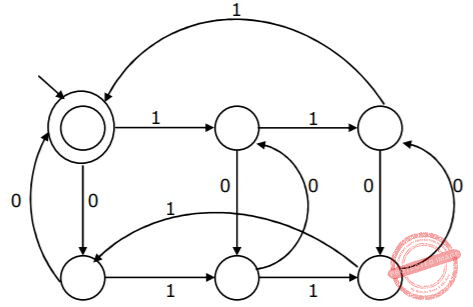A divisible by 3 and 2 B odd and even C even and odd D divisible by 2 and 3
Theory-of-Computation       Finite-Automata
Question 86 Explanation:
Option B:
For example 001 consists of even no. of zero's and odd no. of one's. It is not accepted by TM.
So, it is false.
Option C:
For example 110, contains even 1's and odd 0's but not accepted by TM.
So, it is false.
Option D:
For example 11000, where no. of 1's divisible by '2', and no. of zero's divisible by 3, but not accepted by TM.
So, it is false.
Option A:
It accepts all string where no. of 1's divisible by 3, and no. of zero's divisible by 2.
It is true.
 Question 87

The language {am bn Cm+n | m, n ≥ 1} is

 A regular B context-free but not regular C context sensitive but not context free D type-0 but not context sensitive
Theory-of-Computation       Identify-Class-Language
Question 87 Explanation:
Let us construct a PDA for the language
PUSH z0 into stack
PUSH K to stack of occurrence of a
PUSH L to stack of occurrence of b
POP K and L for the occurrence of c
→ After POP no elements in the stack. So, this is context free language.
Note:
Regular:
R = {an | n ≥ 1}, Example.
CFL:
R = {anbm | n,m ≥ 1}, Example.
 Question 88

Consider the following grammar G:

```S → bS| aA| b
A → bA| aB
B → bB| aS |a ```

Let Na(w) and Nb(w) denote the number of a's and b's in a string w respectively. The language L(G) ⊆ {a, b}+ generated by G is

 A {w|Na(w) > 3Nb(w)} B {w|Nb(w) > 3Na(w)} C {w|Na(w) = 3k, k ∈ {0, 1, 2, ...}} D {w|Nb(w) = 3k, k ∈ {0, 1, 2, ...}}
Compiler-Design       Grammar
Question 88 Explanation:
S→bS
S→baA
S→babA
S→babaB
S→babaa
n(a)=3; n(b)=2
Option A:
Na(w) > 3Nb(w)
3 > 3(2)
3 > 6 (✖️)
Option B:
Nb(w) > 3Nb(w)
2 > 3(2)
2 > 6 (✖️)
Option D:
Nb(w) = 3k
2 = 3k(✖️)
S = aA
S→aA
S→abA
S→abaB
S→abaa
n(a)=3
|n(a)|=3
→ Answer: Option C(✔️)
 Question 89

L1 is a recursively enumerable language over Σ. An algorithm A effectively enumerates its words as w1, w2, w3, ... Define another language L2 over Σ Union {#} as {wi # wj : wi, wj ∈ L1, i < j}. Here # is a new symbol. Consider the following assertions.

```S1: L1 is recursive implies L2 is recursive
S2: L2 is recursive implies L1 is recursive```

Which of the following statements is true?

 A Both S1 and S2 are true B S1 is true but S2 is not necessarily true C S2 is true but S1 is not necessarily true D Neither is necessarily true
Theory-of-Computation       Recursive-Enumerable-Languages
Question 89 Explanation:
Given that
L1 = recursively enumerable language
L2 over Σ∪{#} as {wi#wj | wi, wj ∈ L1, i < j}
S1 is True.
If L1 is recursive then L2 is also recursive. Because, to check w = wi#wj belongs to L2, and we give wi and wj to the corresponding decider L1, if both are to be accepted, then w∈L1 and not otherwise.
S2 is also True:
With the corresponding decider L2 we need to make decide L1.
 Question 90

Choose the best matching between the programming styles in Group 1 and their characteristics in Group 2.

```      Group-1		        Group-2
P. Functional	      1. Command-based, procedural
Q. Logic	      2. Imperative, abstract data type
R. Object-oriented   3. Side-effect free, declarative, expression evaluation
S. Imperative	      4. Declarative, clausal representation, theorem proving ```
 A P - 2, Q - 3, R - 4, S - 1 B P - 4, Q - 3, R - 2, S - 1 C P - 3, Q - 4, R - 1, S - 2 D P - 3, Q - 4, R - 2, S - 1
Programming       Match-the-Following
Question 90 Explanation:
P) Functional programming is declarative in nature, involves expression evaluation and side effect free.
Q) Logic is also declarative but involves theorem proving.
R) Object oriented is imperative statement based and have abstract data types.
S) Imperative programs are made giving commands and follow definite procedure.
There are 90 questions to complete.
PHP Code Snippets Powered By : XYZScripts.com
error: Content is protected !!